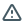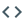# rand

package standard library
Version: go1.19.1 Latest LatestPublished: Sep 6, 2022 License: BSD-3-Clause

##Documentation ¶

### Overview ¶

Package rand implements pseudo-random number generators unsuitable for security-sensitive work.

Random numbers are generated by a Source. Top-level functions, such as Float64 and Int, use a default shared Source that produces a deterministic sequence of values each time a program is run. Use the Seed function to initialize the default Source if different behavior is required for each run. The default Source is safe for concurrent use by multiple goroutines, but Sources created by NewSource are not.

This package's outputs might be easily predictable regardless of how it's seeded. For random numbers suitable for security-sensitive work, see the crypto/rand package.

Example
```Output:

Magic 8-Ball says: As I see it yes
```
Example (Rand)

This example shows the use of each of the methods on a *Rand. The use of the global functions is the same, without the receiver.

```Output:

Float32     0.2635776           0.6358173           0.6718283
Float64     0.628605430454327   0.4504798828572669  0.9562755949377957
ExpFloat64  0.3362240648200941  1.4256072328483647  0.24354758816173044
NormFloat64 0.17233959114940064 1.577014951434847   0.04259129641113857
Int31       1501292890          1486668269          182840835
Int63       3546343826724305832 5724354148158589552 5239846799706671610
Uint32      2760229429          296659907           1922395059
Intn(10)    1                   2                   5
Int31n(10)  4                   7                   8
Int63n(10)  7                   6                   3
Perm        [1 4 2 3 0]         [4 2 1 3 0]         [1 2 4 0 3]
```

### Constants ¶

This section is empty.

### Variables ¶

This section is empty.

### Functions ¶

#### func ExpFloat64 ¶

`func ExpFloat64() float64`

ExpFloat64 returns an exponentially distributed float64 in the range (0, +math.MaxFloat64] with an exponential distribution whose rate parameter (lambda) is 1 and whose mean is 1/lambda (1) from the default Source. To produce a distribution with a different rate parameter, callers can adjust the output using:

```sample = ExpFloat64() / desiredRateParameter
```

#### func Float32 ¶

`func Float32() float32`

Float32 returns, as a float32, a pseudo-random number in the half-open interval [0.0,1.0) from the default Source.

#### func Float64 ¶

`func Float64() float64`

Float64 returns, as a float64, a pseudo-random number in the half-open interval [0.0,1.0) from the default Source.

#### func Int ¶

`func Int() int`

Int returns a non-negative pseudo-random int from the default Source.

#### func Int31 ¶

`func Int31() int32`

Int31 returns a non-negative pseudo-random 31-bit integer as an int32 from the default Source.

#### func Int31n ¶

`func Int31n(n int32) int32`

Int31n returns, as an int32, a non-negative pseudo-random number in the half-open interval [0,n) from the default Source. It panics if n <= 0.

#### func Int63 ¶

`func Int63() int64`

Int63 returns a non-negative pseudo-random 63-bit integer as an int64 from the default Source.

#### func Int63n ¶

`func Int63n(n int64) int64`

Int63n returns, as an int64, a non-negative pseudo-random number in the half-open interval [0,n) from the default Source. It panics if n <= 0.

#### func Intn ¶

`func Intn(n int) int`

Intn returns, as an int, a non-negative pseudo-random number in the half-open interval [0,n) from the default Source. It panics if n <= 0.

Example
```Output:

42
76
30
```

#### func NormFloat64 ¶

`func NormFloat64() float64`

NormFloat64 returns a normally distributed float64 in the range [-math.MaxFloat64, +math.MaxFloat64] with standard normal distribution (mean = 0, stddev = 1) from the default Source. To produce a different normal distribution, callers can adjust the output using:

```sample = NormFloat64() * desiredStdDev + desiredMean
```

#### func Perm ¶

`func Perm(n int) []int`

Perm returns, as a slice of n ints, a pseudo-random permutation of the integers in the half-open interval [0,n) from the default Source.

Example
```Output:

1
2
0
```

`func Read(p []byte) (n int, err error)`

Read generates len(p) random bytes from the default Source and writes them into p. It always returns len(p) and a nil error. Read, unlike the Rand.Read method, is safe for concurrent use.

#### func Seed ¶

`func Seed(seed int64)`

Seed uses the provided seed value to initialize the default Source to a deterministic state. If Seed is not called, the generator behaves as if seeded by Seed(1). Seed values that have the same remainder when divided by 2³¹-1 generate the same pseudo-random sequence. Seed, unlike the Rand.Seed method, is safe for concurrent use.

#### func Shuffle ¶ added in go1.10

`func Shuffle(n int, swap func(i, j int))`

Shuffle pseudo-randomizes the order of elements using the default Source. n is the number of elements. Shuffle panics if n < 0. swap swaps the elements with indexes i and j.

Example
```Output:

[mouth my the of runs corners from ink]
```
Example (SlicesInUnison)
```Output:

C: 3
D: 4
A: 1
E: 5
B: 2
```

#### func Uint32 ¶

`func Uint32() uint32`

Uint32 returns a pseudo-random 32-bit value as a uint32 from the default Source.

#### func Uint64 ¶ added in go1.8

`func Uint64() uint64`

Uint64 returns a pseudo-random 64-bit value as a uint64 from the default Source.

### Types ¶

#### type Rand ¶

```type Rand struct {
// contains filtered or unexported fields
}```

A Rand is a source of random numbers.

#### func New ¶

`func New(src Source) *Rand`

New returns a new Rand that uses random values from src to generate other random values.

#### func (*Rand) ExpFloat64 ¶

`func (r *Rand) ExpFloat64() float64`

ExpFloat64 returns an exponentially distributed float64 in the range (0, +math.MaxFloat64] with an exponential distribution whose rate parameter (lambda) is 1 and whose mean is 1/lambda (1). To produce a distribution with a different rate parameter, callers can adjust the output using:

```sample = ExpFloat64() / desiredRateParameter
```

#### func (*Rand) Float32 ¶

`func (r *Rand) Float32() float32`

Float32 returns, as a float32, a pseudo-random number in the half-open interval [0.0,1.0).

#### func (*Rand) Float64 ¶

`func (r *Rand) Float64() float64`

Float64 returns, as a float64, a pseudo-random number in the half-open interval [0.0,1.0).

#### func (*Rand) Int ¶

`func (r *Rand) Int() int`

Int returns a non-negative pseudo-random int.

#### func (*Rand) Int31 ¶

`func (r *Rand) Int31() int32`

Int31 returns a non-negative pseudo-random 31-bit integer as an int32.

#### func (*Rand) Int31n ¶

`func (r *Rand) Int31n(n int32) int32`

Int31n returns, as an int32, a non-negative pseudo-random number in the half-open interval [0,n). It panics if n <= 0.

#### func (*Rand) Int63 ¶

`func (r *Rand) Int63() int64`

Int63 returns a non-negative pseudo-random 63-bit integer as an int64.

#### func (*Rand) Int63n ¶

`func (r *Rand) Int63n(n int64) int64`

Int63n returns, as an int64, a non-negative pseudo-random number in the half-open interval [0,n). It panics if n <= 0.

#### func (*Rand) Intn ¶

`func (r *Rand) Intn(n int) int`

Intn returns, as an int, a non-negative pseudo-random number in the half-open interval [0,n). It panics if n <= 0.

#### func (*Rand) NormFloat64 ¶

`func (r *Rand) NormFloat64() float64`

NormFloat64 returns a normally distributed float64 in the range -math.MaxFloat64 through +math.MaxFloat64 inclusive, with standard normal distribution (mean = 0, stddev = 1). To produce a different normal distribution, callers can adjust the output using:

```sample = NormFloat64() * desiredStdDev + desiredMean
```

#### func (*Rand) Perm ¶

`func (r *Rand) Perm(n int) []int`

Perm returns, as a slice of n ints, a pseudo-random permutation of the integers in the half-open interval [0,n).

`func (r *Rand) Read(p []byte) (n int, err error)`

Read generates len(p) random bytes and writes them into p. It always returns len(p) and a nil error. Read should not be called concurrently with any other Rand method.

#### func (*Rand) Seed ¶

`func (r *Rand) Seed(seed int64)`

Seed uses the provided seed value to initialize the generator to a deterministic state. Seed should not be called concurrently with any other Rand method.

#### func (*Rand) Shuffle ¶ added in go1.10

`func (r *Rand) Shuffle(n int, swap func(i, j int))`

Shuffle pseudo-randomizes the order of elements. n is the number of elements. Shuffle panics if n < 0. swap swaps the elements with indexes i and j.

#### func (*Rand) Uint32 ¶

`func (r *Rand) Uint32() uint32`

Uint32 returns a pseudo-random 32-bit value as a uint32.

#### func (*Rand) Uint64 ¶ added in go1.8

`func (r *Rand) Uint64() uint64`

Uint64 returns a pseudo-random 64-bit value as a uint64.

#### type Source ¶

```type Source interface {
Int63() int64
Seed(seed int64)
}```

A Source represents a source of uniformly-distributed pseudo-random int64 values in the range [0, 1<<63).

#### func NewSource ¶

`func NewSource(seed int64) Source`

NewSource returns a new pseudo-random Source seeded with the given value. Unlike the default Source used by top-level functions, this source is not safe for concurrent use by multiple goroutines.

#### type Source64 ¶ added in go1.8

```type Source64 interface {
Source
Uint64() uint64
}```

A Source64 is a Source that can also generate uniformly-distributed pseudo-random uint64 values in the range [0, 1<<64) directly. If a Rand r's underlying Source s implements Source64, then r.Uint64 returns the result of one call to s.Uint64 instead of making two calls to s.Int63.

#### type Zipf ¶

```type Zipf struct {
// contains filtered or unexported fields
}```

A Zipf generates Zipf distributed variates.

#### func NewZipf ¶

`func NewZipf(r *Rand, s float64, v float64, imax uint64) *Zipf`

NewZipf returns a Zipf variate generator. The generator generates values k ∈ [0, imax] such that P(k) is proportional to (v + k) ** (-s). Requirements: s > 1 and v >= 1.

#### func (*Zipf) Uint64 ¶

`func (z *Zipf) Uint64() uint64`

Uint64 returns a value drawn from the Zipf distribution described by the Zipf object.

## Cached: rand package - math/rand - Go Packages

https://pkg.go.dev/math/rand

This is a cached page of the above url, please refer to the original page if it is not offline.

Snapshot was generated on 22 September 2022. (2022/09/22 at 16:07:19 +0000 UTC)

MrWaggel.be